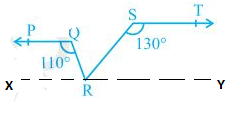Guru

# In Fig. 6.31, if PQ ST, PQR = 110° and RST = 130°, find QRS. [Hint : Draw a line parallel to ST through point R.] Q.4

• 0

Please give me the best way for solving the problem of class 9th ncert math of Lines and Angles chapter of math of class 9th of exercise 6.2of question no 4 what is the tricky way for solving this question In Fig. 6.31, if PQ ST, PQR = 110° and RST = 130°, find QRS. [Hint : Draw a line parallel to ST through point R.]

Share

1. First, construct a line XY parallel to PQ.We know that the angles on the same side of transversal is equal to 180°.

So, PQR+QRX = 180°

Or,QRX = 180°-110°

∴ QRX = 70°

Similarly,

RST +SRY = 180°

Or, SRY = 180°- 130°

∴ SRY = 50°

Now, for the linear pairs on the line XY-

QRX+QRS+SRY = 180°

Putting their respective values, we get,

QRS = 180° – 70° – 50°

Hence, QRS = 60°

• 0